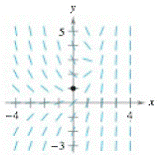Chapter 6.4, Problem 15E

Chapter
Section
Textbook Problem

Slope Field In Exercises 15 and 16, (a) sketch an approximate solution or the differential equation satisfying the given initial condition on the slope field, (b) find the particular solution that satisfies the given initial condition, and (c) use a graphing utility to graph the particular solution. Compare the graph with the sketch in part (a). To print an enlarged copy of the graph, go to MaihGraphs.com. d y d x = e x − y(a)

To determine

To graph: An approximate solution of the differential equation, dydx=exy, satisfying the initial condition, (0,1) on the slope field.

Explanation

Given:

The differential equation is, dydx=exy, the initial condition is, (0,1), and the slope field is shown below,

Graph:

Consider the given differential equation,

dydx=exy

Make a table for the slopes at different points as shown below,

 x −2 −2 −1 −1 0 0 1 1 2 2 y 1 −1 1 −1 1 −1 1

(b)

To determine
The particular solution of the differential equation, dydx=exy, satisfying the initial condition, (0,1) on the slope field.

(c)

To determine

To graph: The particular solution, y=12(ex+ex), using graphing utility and compare the graph with the graph obtained in part (a).

Still sussing out bartleby?

Check out a sample textbook solution.

See a sample solution

The Solution to Your Study Problems

Bartleby provides explanations to thousands of textbook problems written by our experts, many with advanced degrees!

Get Started

Solve the equations in Exercises 112 for x (mentally, if possible). x+5=0

Finite Mathematics and Applied Calculus (MindTap Course List)

2. Define the terms population, sample, parameter and statistic.

Statistics for The Behavioral Sciences (MindTap Course List)

Explain why 2004 nickels are worth more than 100.

Mathematical Excursions (MindTap Course List)